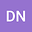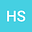loading page

Global well-posedness and optimal time decay rates of solutions to the three-dimensional magneto-micropolar fluid equations
••Haifeng Shang
Henan Polytechnic University
Author Profile## Abstract

This paper deals with the global existence and decay estimates of solutions to the three-dimensional magneto-micropolar fluid equations with only velocity dissipation and magnetic diffusion in the whole space with various Sobolev and Besov spaces. Specifically, we first investigate the global existence and optimal decay estimates of weak solutions. Then we prove the global existence of solutions with small initial data in $H^s$, $B_{2, \infty}^s$ and critical Besov spaces, respectively. Furthermore, the optimal decay rates of these global solutions are correspondingly established in $\dot{H}^m$ and $\dot{B}_{2, \infty}^m$ spaces with $0\leq m\leq s$ and in $\dot{B}_{2, 1}^{m}$ with $0\leq m\leq \frac 12$, when the initial data belongs to $\dot{B}_{2, \infty}^{-l}$ ($0< l\leq\frac32$). The main difficulties lie in the presence of linear terms and the lack of micro-rotation velocity dissipation. To overcome them, we make full use of the special structure of the system and employ various techniques involved with the energy methods, the improved Fourier splitting, Fourier analysis and the regularity interpolation methods.